﻿ 手绘路径下两轮智能车控制方法设计与实现 Control Method & Implementation of Two-Wheel Smart Car under Hand-Drawn Route

Computer Science and Application
Vol. 09  No. 05 ( 2019 ), Article ID: 30253 , 7 pages
10.12677/CSA.2019.95099

Control Method & Implementation of Two-Wheel Smart Car under Hand-Drawn Route

Desheng Zhang1*, Bin Sun1,2, Kui Chen1,3, Shun Wang1, Jingzhi Qu4

1Xuzhou University of Technology, Xuzhou Jiangsu

2China University of Mining and Technology, Xuzhou Jiangsu

3Jiangsu Key Laboratory of Large Engineering Equipment Detection and Control, Xuzhou Jiangsu

4Jiangsu ZKCH Information & Technology Co., Ltd., Xuzhou Jiangsu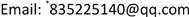Received: Apr. 30th, 2019; accepted: May 10th, 2019; published: May 17th, 2019ABSTRACT

In handling, patrol and other work scenarios, considering the complexity and changeability of workplace layout deployment, a method of generating trolley movement path control coordinates and batch generation of trolley control parameters by free hand-painting is proposed in this paper. The hand-drawn paths, parameter extraction and optimization, Back-stepping control parameter solving and wireless batch download be executed on the PC using LabVIEW. At car side, the control parameters be periodically read and executed in open-loop mode. The experiments show that the hand-painted method and the tracking control strategy designed in this paper are correct and effective, and the trolley travels according to the hand-drawn path, and its error meets the requirements.

Keywords:Hand-Drawn Route, Path Tracking, Motion Control Strategy

1徐州工程学院，江苏 徐州

2中国矿业大学，江苏 徐州

3江苏省大型工程装备检测与控制重点建设实验室，江苏 徐州

4江苏中矿创慧科技有限责任公司，江苏 徐州1. 引言

2. 系统总体流程

3. 路径生成

3.1. 手绘路径线条方法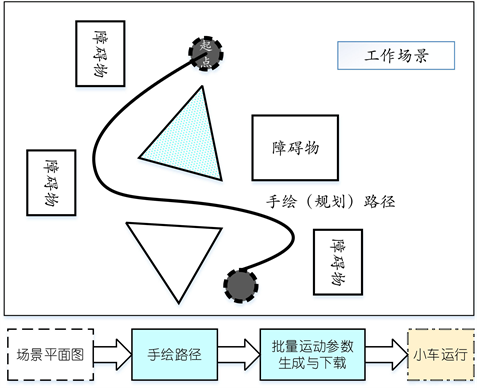Figure 1. System working principle and processFigure 2. Hand-drawn curves and hand-drawn curve coordinates

3.2. 路径像素点抽取与优化

4. 轨迹控制参数设计

4.1. 小车运动学分析  - 

${v}_{c}=\frac{R}{2}\left({\omega }_{r}+{\omega }_{l}\right),\text{\hspace{0.17em}}{\omega }_{c}=\frac{R}{D}\left({\omega }_{r}-{\omega }_{l}\right)$

${v}_{c}$${\omega }_{c}$ 分别为机器人直线运动速度和旋转角速度； ${\omega }_{r}$${\omega }_{l}$ 分别为机器人小车两轮速度；R为车轮半径；D为两轮间距。其位置姿态微分方程可以描述如下：

$\left[{\stackrel{˙}{x}}_{c},{\stackrel{˙}{y}}_{c},{\stackrel{˙}{\theta }}_{c}\right]=\left[{v}_{c},{\omega }_{c}\right]\left[\begin{array}{ccc}\mathrm{cos}{\theta }_{c}& \mathrm{sin}{\theta }_{c}& 0\\ 0& 0& 1\end{array}\right]$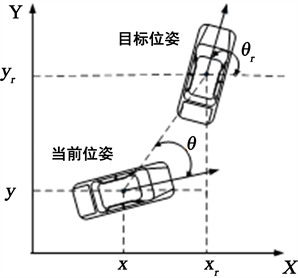Figure 3. 2-wheeled car 2-freedom kinematics model

${p}_{e}=\left[\begin{array}{c}{x}_{e}\\ {y}_{e}\\ {z}_{e}\end{array}\right]=\left[\begin{array}{c}\omega {y}_{e}-v+{v}_{r}\mathrm{cos}{\theta }_{e}\\ -\omega {x}_{e}+{v}_{r}\mathrm{sin}{\theta }_{e}\\ {\omega }_{r}-\omega \end{array}\right]$

$\underset{t\to \infty }{\mathrm{lim}}\left[|{x}_{e}\left(t\right)|+|{y}_{e}\left(t\right)|+|{\theta }_{e}\left(t\right)|\right]=0$

4.2. 小车运动控制设计

$\left[{\omega }_{r},{\omega }_{l}\right]$ ，即转角值。控制器的定时中断时长设定为 $\Delta t=100\text{\hspace{0.17em}}\text{ms}$ ，即每100 ms读取一次接收到的 $\left[{\omega }_{r},{\omega }_{l}\right]$

4.3. 运动参数通信数据包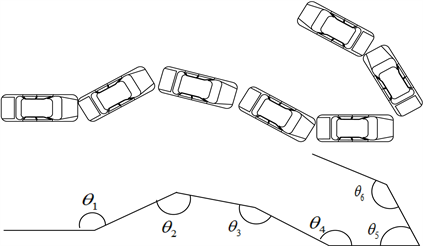Figure 4. Motion control strategy for car trail tracking

5. 实验与测试

PC端自由手绘出“S”与“Z”形路径，再利用Back-stepping方法解算出各时刻 $\Delta t$ 的控制参数 $\left[{\omega }_{r},{\omega }_{l}\right]$ ，按照串口通信数据规则形成批量的参数一次性下载到小车存储器内。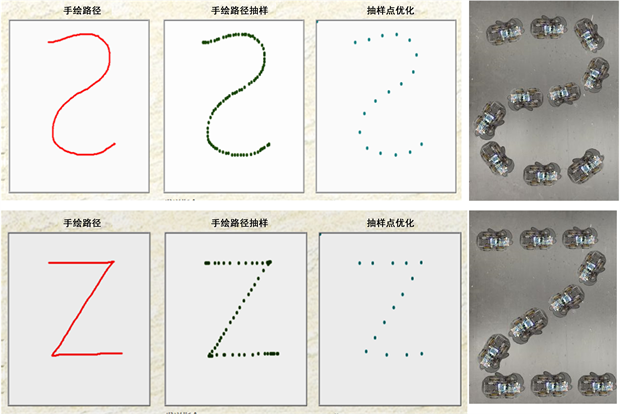Figure 5. Hand-drawn path and actual driving path of the carTable 2. Measured accuracy and image positioning (cm)

6. 结语

1) 江苏省科技项目(BY2015024-03)城市施工环境扬尘污染监测与预警系统研发；2) 徐州市科技计划工业科技项目(KC15GM045)资助；3) 江苏省大型工程装备检测与控制重点建设实验室开放基金(JSKLEDC201414)项目资助。

Control Method & Implementation of Two-Wheel Smart Car under Hand-Drawn Route[J]. 计算机科学与应用, 2019, 09(05): 883-889. https://doi.org/10.12677/CSA.2019.95099

1. 1. 蔺宏良, 黄晓鹏. 车联网技术研究综述[J]. 机电工程, 2014(9): 1235-1238.

2. 2. 史晨红, 等. 基于轨迹控制的AGV运动控制器设计研究[J]. 机械设计与制造工程, 2014(2): 7-12.

3. 3. 李美花, 等. 微传感器阵列多通道数据采集和处理系统[J]. 电子测量与仪器学报, 2016(2): 311-317.

4. 4. 符晓勇. 智能小车软件系统设计与路径规划方法研究[D]: [硕士学位论文]. 杭州: 浙江理工大学, 2017.

5. 5. 柳胜凯, 等. 基于多传感器数据融合的室内行人航位推算法[J]. 传感器与微系统, 2018(3): 133-137.

6. 6. 蔡晓慧. 基于智能算法的移动机器人路径规划研究[D]: [硕士学位论文]. 杭州: 浙江大学, 2007.

7. 7. 王宇, 等. 一种新的智能小车控制策略[J]. 浙江工业大学学报, 2012(2): 217-221.

8. 8. 姜立标, 吴中伟. 基于趋近律滑模控制的智能车辆轨迹跟踪研究[J]. 农业机械学报, 2018, 49(3): 381-387.

9. 9. 杨李朋, 张文丰, 王昊. 两轮差速AGV的轨迹跟踪控制研究[J]. 软件工程与应用, 2017, 6(4): 59-67.

10. 10. 王盼宝. 智能车制作: 从元器件、机电系统、控制算法到完整的智能车设计[M]. 北京: 清华大学出版社, 2017.

11. 11. 黄英豪, 黎兆宇. 汽车坐标系和车身姿态角的确定方法[J]. 装备制造技术, 2012(10): 113-115.

12. 12. 郭烈, 黄晓慧, 葛平淑, 等. 基于反演法的智能车辆弯路换道轨迹跟踪控制[J]. 吉林大学学报(工版), 2013, 43(2): 323-328.

13. 13. 张扬名, 刘国荣, 兰永红. 一种改进的移动机器人轨迹跟踪迭代学习方法[J]. 计算机应用研究, 2012, 29(10): 3728-3732.

14. 14. 李渊, 马戎, 付维平. 智能车辆的滑模轨迹跟踪控制[J]. 测控技术, 2012, 31(9): 71-74.

NOTES

*通讯作者。